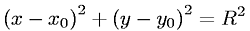Equations > Geometry > Curves and Shapes > Equation of a Circle

### Equation of a CircleLatex Code:

MathML Code:

 ${\left(x-{x}_{0}\right)}^{2}+{\left(y-{y}_{0}\right)}^{2}={R}^{2}$

MathType 5.0: